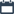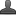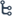## Tuesday, October 27, 2020

•October 27, 2020
••, ,
•1 comment

## Important questions for 10th class Maths chapter-7 Coordinate Geometry,Mathematics questionnaire-x class

7. COORDINATE GEOMETRY

Hi friends and my dear students! In this post, I have covered Important questions for 10th class maths chapter-7 Coordinate Geometry, Chapter wise previous year Questions. After Reading  Please do share it with your friends. Learn maths for All classes here

PRIORITY-I

1 MARK QUESTIONS

1. What is the distance between A (4, 0) and B (8, 0)?

2. Find the slope of the line passing through the points A(0,4) and B(4,0)

3. What is the distance between A (8, 3) and B (-4, 3)?

4. Find the distance between the points origin and A (7, 4)?

5. Find the distance between A (2, 0) and B (0, 4)?

6. Find the distance between A (4, 2) and B (8, 6)?

7. Find the midpoint of line segment joining the points (3, 0) and (1, -4)?

8. Find the centroid of the triangle whose vertices are (3, -5), (-7, 4) and (10, -2)?

9. The points (2, 3), (x, y) and (3,-2) are vertices of a triangle. If the centroid of this triangle is again(x, y), find (x, y)?

10. The end points of line are (2, 3) and (4, 5). Find the slope of the line?

11. Find the slope of the line AB with A (4, -6) and B (7, 2)?

2 MARKS QUESTIONS

12. Find the distance between A (2, 3) and B(4,1)?

13. If the distance between (2,-3) and (10,y) is 10 units then find y

14. Name the type of quadrilateral formed by the points(-1,-2),(1,0), (-1,2), (-3,0) and give reasons for your answer

15. Find the centroid of the triangle formed by the line 4x+5y-20=0 with co – ordinate axis?

16. In what ratio does the point (-4,6) divide3s the line segment joining the points A(-6,10) and B(3,-8)

17. Find the point on x-axis which is equidistant from (2,-5) and (-2, 9)?

18. If the distance between two points (x, 7) and (1, 15) is 10. Find x?

19. Find the radius of the circle whose Centre is (3, 2) and passes through (-5,6)?

20. Find the coordinates of the point which divides the line segment joining the points (4,-3) and (8, 5) in the ratio 3:1 internally?

21. In what ratio does the point (-4,6) divide the line segment joining the points A(-6,10) and B(3,-8)?

22. Find the ratio in which the y-axis divide the line segment joining the points (5,-6) and (1,-4). Also find the point of intersection?

23. Find the area of triangle whose vertices are (1,-1), (-4, 6) and (-3,-5)?

24. Find the area of triangle formed by the points A (5, 2), B (4, 7) and C (7, -4)?

25. The points (3,-2), (-2,8) and (0,4) are three points in a plane. Show that these points are collinear?

26. Find the value of b for the points (1, 2) (-1, b) and (-3, -4) are collinear?

27. Determine the x so that 2 is the slope of the line through P (2, 5) and Q (x, 3)?

Also Check

Important questions for 10th class maths chapter-1 Real numbers

Important questions for 10th class maths chapter-4 LINEAR EQUATIONS

Important questions for 10th class maths chapter-3 polynomial

4 MARKS QUESTIONS:

28. Show that the points A (4, 2), B (7, 5), C (9, 7) are lie on a same plane?

29. Find the coordinates of points which divide the line segment joining A(-4,0) and B(0,6) into four equal

30. Show that the points (1, 7), (4, 2), (-1, -1) and (-4, 4) are vertices of square?

31. Find a relation between x and y such that the point (x, y) is equidistant from the points (7, 1) and (3, 5)?

32. Find a point on the y-axis which is equidistant from the points A (6, 5) and B (-4, 3)?

33. Verify the points (1, 5), (2, 3) and (-2, -1) are collinear or not?

34. Show that the points A (a, o), B (-a, o), C (0, a) are form an equilateral triangle?

35. Show that the points (-4, -7), (-1, 2), (8, 5) and (5,-4) are vertices of rhombus?

36. Find the coordinates of the points of trisection of the line segment joining the points A (2, -2) and B (-7, 4)?

37. Find the coordinates of the points of trisection of the line segment joining the points A (2, 6) and B (-4, 8)?

38. Show that the points (7, 3), (6, 1), (8, 2) and (9, 4) are vertices of parallelogram.?

39. If the points A( 6,1),B (8,2), C(9,4) and D(p,3) are the vertices of a parallelogram, find p?

40. If A(-5,7), B(-4,-5),C(-1,-6) and D(4,5) are the vertices of a quadrilateral ,then find the area of quadrilateral ABCD?

41. Find the area of a triangle whose lengths of sides are 15m, 17m, 21m, use Heron’s formula and verify your answer by using the formula A= ½ bh?

42. Find the area of a triangle formed by the points (0,0),(4,0),(4,3) by using Heron’s formula?

43. Find the area of a triangle formed by joining midpoints of the sides of the triangle whose vertices are (0,-1), (2,1),and (0,3). Find the ratio of this area to the area of the given triangle?

44. Find the area of quadrilateral whose vertices are (-4,-2),(-3,-5),(3,-2) and (2,3)?

45. Find the area of a triangle formed by the points (2,3),(-1,3),(2,-1) by using Heron’s formula?

PRIORITY II:

1 MARK QUESTIONS:

1. Find the slope of line joining (2,3) and (2,6)

2. If the line 2x+3y=k passes through origin, find ‘k’

3. Find midpoint of points(2,7) and (12,-7)

4. Write the co-ordinates of p which divides the line segment joining A(x1,y1) and B(x2,y2) in the ratio m1:m2

5. Find the slope of line perpendicular to 2x+3y+5=0

6. Which points are called collinear points?

7. Write area of triangle using heron’s formulae

8. What do you meant by trisectional points of a line?

9. A,B and C are any three points such that AB+BC=AC what can you say about the points

10. Name quadrant to which the following points belongs (-3,7),(8,1)

12. Find perpendicular distance of A(5,12) from y-axis

13. If C(2,p) is a point on the line segment joining the points A(6,5) and B(2,11) explain condition for the point ‘C’ to become the midpoint of AB

14. Which of the points Q(3,5) and R(-1,1) are nearer to the point p(4,4)

15. Write the distance between A(a+b,a-b),B(a-b, a+b)

16. Write the formulae to find distance between two points on the same horizontal line and vertical line

17. Mention the coordinate axis on which the points (0,6),(0,-2),(0, √2)

18. Find the distance between the point p(cosα , -sinα )and Q(-cosα , sinα )

19. (3,-4) is a point on a circle whose center is origin. Guess the radius of the circle.

2MARKS QUESTIONS:

20. If p=(2,5), Q=(x,-7) find the possible value of x so that PQ=13

21. Find the distance between points(acosx,0) and (0,asinx)

22. Find are area of triangle formed by vertices(3,0), (0,4), (0,0)

23. In what ratio is segment joining the points (-3,2) and(6,1) divide by y-axis

24. If p(b+c,c+a) and Q(c+a,a+b) find PQ

25. An ant is at (4,5) on graph sheet mounted of a wall. If it moves to a point (5,2) and turns to reach another point (3,6) find the distance travelled by the ant.

26. Find the coordinates of the centroid of the triangle, whose vertices are(-4,4), (-2,2) and(6,12)

27. Find the coordinates of the centre of circle having the points (9,3) and (1,-1) as the end points of the diameter

28. If you plot the points (0,0), (2,0), (2,2), (0,2) and join them in order. Name the quadrilateral so obtained based on your observations

4 MARKS QUESTIONS:

1. Vertices of a triangle ABC are A(3,5), B(7,4) and C(10,8). The midpoints of the sides BC,CA and AB are D,E and F respectively are the centroid of and are same or not

2. If the distances of p(x,y) from A(a+b,b-a) and B(a-b,a+b) are equal, then prove that a / b=x/ y

3. If p(x,y) is any point on the line joining the points A(a,0) and B(0,b) then show that x/a+y/b=1

4. Prove that the area of triangle whose3 vertices are(t,t-2),(t+2,t+2) and (t+3,t) is independent of t

5. Prove that the midpoint of the hypotenuse of a right angled triangle is equidistant from its vertices.

6. The line joining points A(6,9) and B(-6,-9) are given

i. in which ratio does origin divide AB? And what it is called for AB ?

ii.in which ratio does the point P(2,3) divide AB? iii.in which ratio does the point q(-2,-3) divide AB

iv. In how many equal parts is AB divided by P and Q

v. what do we call P and Q for AB?

#### 1 comment:

1.This is one of the best blogs I've come across recently to study Maths. I appreciate the author's efforts in writing these educational articles. Thank you for sharing this. Great blog. Pearson Maths Past Papers